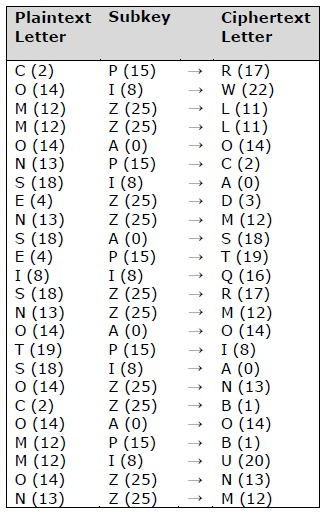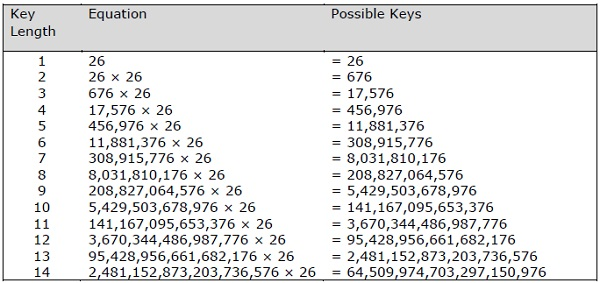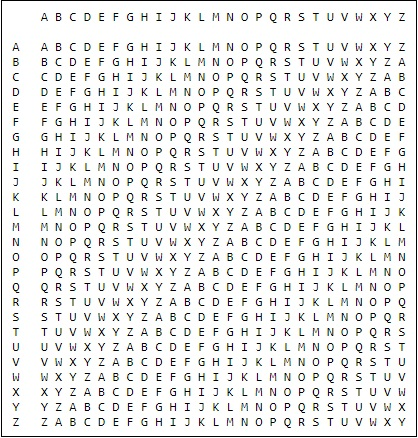# Understanding Vignere Cipher

Vignere Cipher includes a twist with Caesar Cipher algorithm used for encryption and decryption. Vignere Cipher works similar to Caesar Cipher algorithm with only one major distinction: Caesar Cipher includes algorithm for one-character shift, whereas Vignere Cipher includes key with multiple alphabets shift.

## Mathematical Equation

For encryption the mathematical equation is as follows −

Ek(Mi)=(Mi+Ki)mod26

${E}_{k}\left(M{}_{i}\right)=\left({M}_{i}+{K}_{i}\right)\phantom{\rule{thickmathspace}{0ex}}\phantom{\rule{thickmathspace}{0ex}}\phantom{\rule{thickmathspace}{0ex}}mod\phantom{\rule{thickmathspace}{0ex}}\phantom{\rule{thickmathspace}{0ex}}26$

For decryption the mathematical equation is as follows −

Dk(Ci)=(CiKi)mod26

${D}_{k}\left(C{}_{i}\right)=\left({C}_{i}-{K}_{i}\right)\phantom{\rule{thickmathspace}{0ex}}\phantom{\rule{thickmathspace}{0ex}}\phantom{\rule{thickmathspace}{0ex}}mod\phantom{\rule{thickmathspace}{0ex}}\phantom{\rule{thickmathspace}{0ex}}26$

Vignere cipher uses more than one set of substitutions, and hence it is also referred as polyalphabetic cipher. Vignere Cipher will use a letter key instead of a numeric key representation: Letter A will be used for key 0, letter B for key 1 and so on. Numbers of the letters before and after encryption process is shown below −The possible combination of number of possible keys based on Vignere key length is given as follows, which gives the result of how secure is Vignere Cipher Algorithm −## Vignere Tableau

The tableau used for Vignere cipher is as shown below −